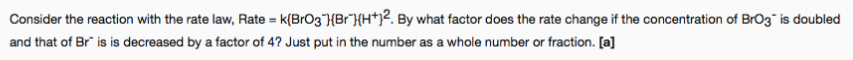# Consider the reaction with the rate law, Rate = k{BrO3^-}{Br^-}{H^+}^2. By what factor does the rate change if the concentration of BrO3^- is doubled and that of Br^- is decreased by a factor of 4? Just put in the number as a whole number or fraction. [a]